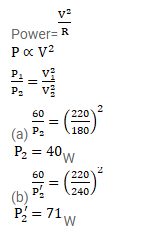# An electric bulb, when connected across a power supply of

Question:

An electric bulb, when connected across a power supply of $220 \mathrm{~V}$, consumes a power of $60 \mathrm{~W}$. If the supply drops to $180 \mathrm{~V}$, what will be the power consumed? If the power supply is suddenly increased to $240 \mathrm{~V}$, what will be the power consumed?

Solution: